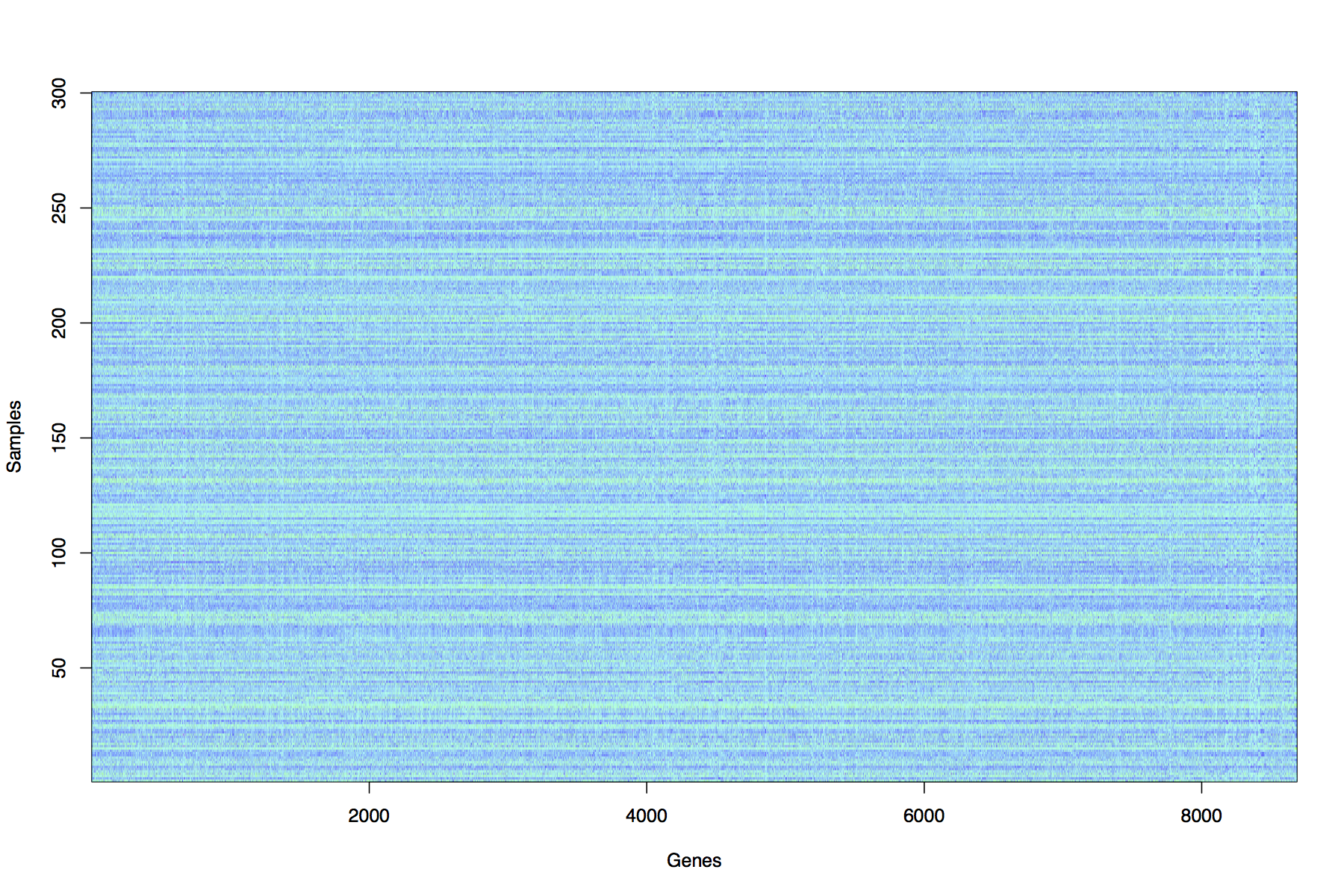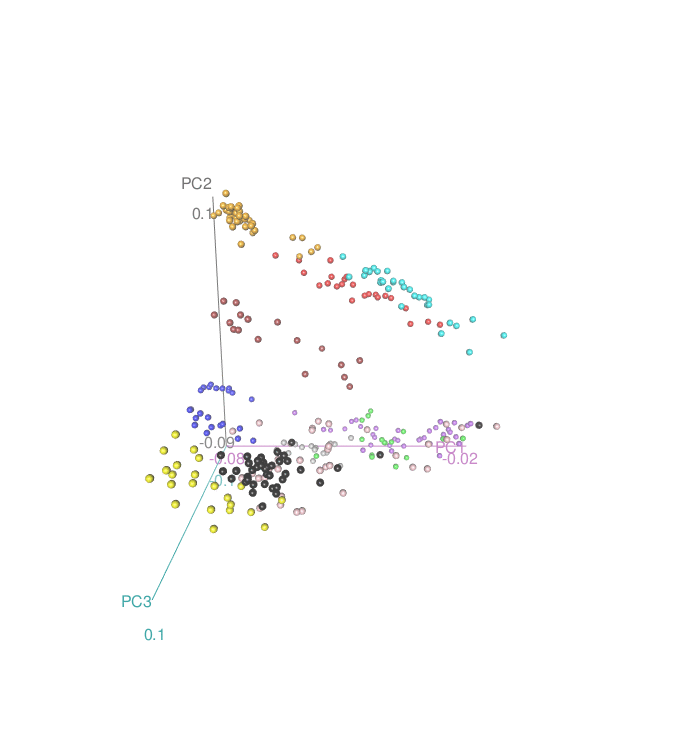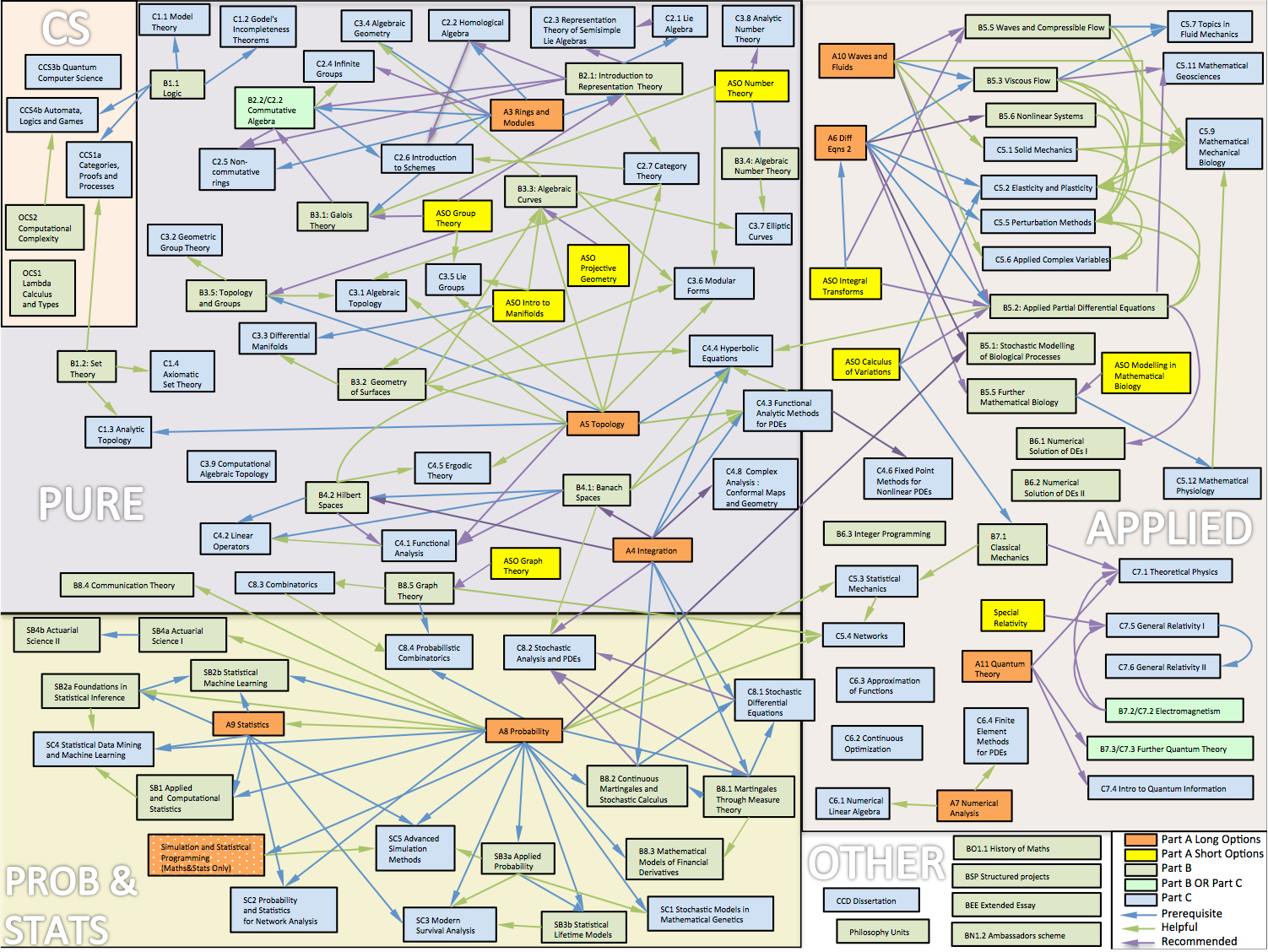## Teaching

This page contains some of the teaching resources I developed while in Oxford.

### Prelims Statistics (Unsupervised Learning)

I developed this course to double the amount of Statistics taught to 1st year Maths/Stats students at Oxford (2016-2017)

The aim of the course is to introduce students to the theory and practice of unsupervised learning.

Unsupervised learning can be described as finding structure in datasets, and has applications in many areas such as finance, retail, medical imaging, sports performance analysis, genetics, medicine, studies of the environment and social networks.

Unsupervised learning methods are important parts of Computational Statistics, Machine Learning, Artificial Intelligence and Big Data.

Motivating example

Raw dataset : 300 x 8686 matrix of gene expression measurements from Pollen et al (2014) Nature Biotechnology 32, 1053-1058 Viewing the raw data it is very difficult to see any clear structure or similarity between the samples.3D Projection and clustering : The method of Principal Components Analysis (PCA) has been applied to the dataset in order to uncover structure. A clustering method (k-means) has then been applied to group observations in distinct groupings or clusters. Students will learn the theory and practical skills to reproduce this analysis.#### Lecture slides and course notes

Students should take notes in each lecture, but I will use slides as visual aids to illustrate various concepts and results. slides.pdf

Here is a link to the course notes course_notes.pdf

#### Exercise sheets

There will be 3 exercise sheets for this part of the course.

Sheet pdf
1 sheet6.pdf
2 sheet7.pdf
3 sheet8.pdf

#### R/Matlab exercises

Each sheet will contain a mix of written questions and Optional questions to be done either using R or Matlab.

It is up to each college tutor to decide whether students should attempt these questions, but it is strongly recommended, as these questions will help with understanding of the theory.

Modern statistics is pervasive in the era of “Big Data”. The majority of Maths graduates will go on to careers that involve some use of data, so a firm practical grounding in statistical analysis is highly valuable. An aim of this course is to get students started on being able to independently carry out statistical data analysis.

As many student will not have worked with R, here is a short tutorial document that will introduce R, show students how to install R and get started with some basics.

R_intro.pdf

##### Book

The following book gives a good overview of the methods covered in this course

This book is freely available online here http://www-bcf.usc.edu/~gareth/ISL/

G. James, D. Witten, T. Hastie, R. Tibshirani An Introduction to Statistical Learning (with Applications in R) (Springer 2013)

Chapter 10 covers unsupervised learning.

### Part B Foundations of Statistical Inference (BS2a)

This was an existing course for 3rd year Maths/Stats students on statistical theory. I amended the syllabus to include material on the EM algorithm and Variational Bayes and approximations to marginal likelihood : Laplace approximation and BIC. (2012-2013)

Course aims Understanding how data can be interpreted in the context of a statistical model. Working knowledge and understanding of key-elements of model-based statistical inference, including awareness of similarities, relationships and differences between Bayesian and frequentist approaches.

Synopsis

Exponential families Curved and linear exponential families; canonical parametrization; likelihood equations. Sufficiency: Factorization theorem; sufficiency in exponential families.

Frequentist estimation unbiasedness; method of moments; the Cramer-Rao information inequality; Rao-Blackwell theorem, Lehmann-Scheffe Theorem and Rao-Blackwellization. Statement of complete sufficiency for Exponential families.

The Bayesian paradigm likelihood principal; subjective probability; prior to posterior analysis; asymptotic normality; conjugacy; examples from exponential families. Choice of prior distribution: proper and improper priors; Jeffreys and maximum entropy priors. Hierarchical Bayes models.

Computational techniques Markov chain Monte Carlo methods; The Metropolis- Hastings algorithm. Gibbs Sampling. Variational Bayesian methods. The EM algorithm. Approximations to marginal likelihood : Laplace approximation and BIC.

Decision theory risk function; Minimax rules, Bayes rules. Point estimators and admissability of Bayes rules. The James-Stein estimator, shrinkage estimators and Empirical Bayes. Hypothesis testing as decision problem.

#### Lecture slides

PDF of all 16 lectures in 4up format : bs2a_4up.pdf

#### Exercise sheets

Class Sheet
Week 3 ex1.pdf
Week 4 ex2.pdf
Week 5 ex3.pdf
Week 6 ex4.pdf
Week 7 ex5.pdf
Week 8 ex6.pdf

P. H. Garthwaite, I. T. Jolliffe and Byron Jones, Statistical Inference, OUP
G.A.Young and R.L. Smith, Essentials of Statistical Inference, CUP.
T. Leonard and J.S.J. Hsu, Bayesian Methods, CUP.

D. R. Cox, Principles of Statistical Inference, CUP
H. Liero and S Zwanzig, Introduction to the Theory of Statistical Inference, CRC Press
D. Barber, Bayes Reasoning and Machine Learning, CUP

### Part A Statistical Programming

I developed this course to introduce statistical computing (using R) to undergraduate Maths&Stats students in the their 2nd year (previously it took until the 3rd year before they had any exposure to statistical computing. (2010-2011)

The aim of the course is to introduce students to how to carry out statistical analysis using a computer and the theory of statistical programming and its related techniques. The course will be based on the R programming language which is now widely used in all branches of applied statistics.

#### Lectures and practical sessions

There will be 6 classes, made up of a lecture followed by a computer practical session (using R): all lectures and all practical sessions are an essential part of the course. If practical sheets are not finished in the practical sessions then they should be completed outside the practical.

Topic Lectures Practical
Introduction week1.pdf week1prac.pdf
Working with data week2.pdf week2prac.pdf
Programming I week3.pdf week3prac.pdf
Programming II week4.pdf week4prac.pdf
Solving Equations and Optimization week5.pdf week5prac.pdf
Simulation week6.pdf week6prac.pdf

Datasets

The following datasets are used in the practical sessions

hellung.txt
cystfibr.txt
juul.txt
AIDS.txt
speed.txt

#### Exercise sheets

As the exam for this course is a written exam it is important to practice questions without using a computer.

For this reason there will be two written exercise sheets with a class for each one. The details are below.

Written exercise sheet Deadline Class times
Week 7 : Sheet1.pdf Tues 8 March, by 5pm, SPR1 Thur 10 March 2-3pm, 3-4pm
Week 8 : Sheet2.pdf Tues 10 May, by 5pm, SPR1 Thur 12 May 2-3pm, 3-4pm

Programming with R The course will teach you how to write programs using the statistical computing environment called R.

If you have your own computer you are strongly encouraged to download and install the software (it’s free) so that you can work on the problem sheets and complete any of the practicals as required.

Books In addition to the extensive documentation and help system that is included in R there are two main books that we recommend.

`Introductory Statistics with R’ by Peter Dalgaard, ISBN 0-387-95475-9

– a very good introduction to R that includes many biostatistical examples and covers most of the basic statistics covered in this course.

`Modern Applied Statistics with S’ by Bill Venables and Brian Ripley, ISBN 0-387-95457-0

– a comprehensive text that details the S-PLUS and R implementation of many statistical methods using real datasets.

### Introduction to Probability and Statistics

this course was taken by 1st year students in Psychology and Human Sciences This lecture course was taught for 1st year Psychology and Human Sciences students (2002-2008)

#### Lecture Slides, Notes and Exercise Sheets

Lecture Topic Notes Slides Exercises
Lecture 1 Plotting Data + Summaries L1.notes.pdf L1.slides.pdf L1.exercise.pdf
Lectures 2+3 Probability L2.notes.pdf L2.slides.pdf L2.exercise.pdf
Lecture 4 Binomial Distribution L4.notes.pdf L4.slides.pdf L4.exercise.pdf
Lecture 5 Poisson Distribution L5.notes.pdf L5.slides.pdf L5.exercise.pdf
Lecture 6 Normal Distribution L6.notes.pdf L6.slides.pdf L6.exercise.pdf
Lecture 7 Hypothesis Tests L7.notes.pdf L7.slides.pdf L7.exercise.pdf
Lecture 8 Chi-squared Tests L8.notes.pdf L8.slides.pdf L8.exercise.pdf

Recommended Books The two books that have been recommended in past years for Psychology and Human Sciences respectively are

HOWELL, David C. Statistical Methods for Psychology Fiffth Edition 802pp Hardback (ISBN 053437770) £26.99

COHEN, Lousi and HOLLIDAY, Michael Practical Statistics for Students 352pp Paperback (ISBN 1853963291) £17.99